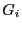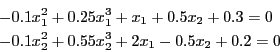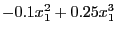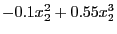Next: The CoeffLinear procedure Up: Implementation without gradient Previous: Implementation without gradient   Contents

The NonLinear procedure

The purpose of the procedure is to compute an interval evaluation of the non linear term of the equations (theterms in the mathematical background). This procedure take as argument a box and the returned evaluation of the non-linear terms. Consider for example the equations:The non-linear terms of these equations areandand the NonLinear procedure is written as:

void NonLinear(INTERVAL_VECTOR &x,INTERVAL_VECTOR &X)
{
X(1) = (-0.1+0.25*x(1))*Sqr(x(1));
X(2) = (-0.1+0.55*x(2))*Sqr(x(2));
}

Jean-Pierre Merlet 2012-12-20